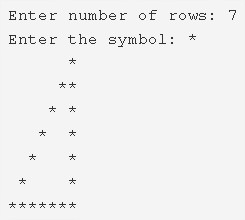# C Program Hollow Mirrored Right Triangle Star Pattern

C Program to print a Hollow mirrored Right Triangle Star Pattern – In this article, we will learn how to print a hollow mirrored right triangle star pattern in various ways in C programming.

Suitable examples and sample programs have also been added so that you can understand the whole thing very clearly. The compiler has also been added with which you can execute it yourself.

The ways mentioned in this piece are as follows:

• Using For Loop
• Using While Loop
• Using Do-While Loop

As we all know, a right triangle is a special type of a triangle where one of the angles is 90 degrees.

The sum of the squares of the adjacent sides to the right angle is equal to the square of the side opposite to the right angle, as per the Pythagoras theorem.As you can see, we have to first enter the number of rows. Depending on that, the right triangle can be printed using the symbol “*”.

Thus, the various methods used to print a hollow mirrored right triangle star pattern are as follows:

## Using For Loop

1) Read the number of rows and store the value into the variables n. Read the entered character using getchar() and store it in the variable ch.

2) The outer for loop iterates through rows from i=1 to n.

3) The 1st inner for loop iterates through columns with the structure for(j=1;j<=n-i;j++) and print space.

4) For 1st row or nth row

a) The 2nd inner for loop iterates through columns with the structure for(j=1;j<=i;j++) and prints symbol.

5) For other than the 1st row, nth row

a) The 3rd inner for loop iterates through columns with the structure for(j=1;j<=i;j++).

b) If j=1 or i , it prints symbol. Otherwise, it prints space.

6) Cursor comes to next line after each iteration of outer for loop.

Output:

### Using While Loop

1) The outer while loop iterates through rows until the condition i<=n becomes false.

2) The 1st inner while loop iterates through columns until the condition j<=n-i.

Prints space, j value increased by 1.

3) For i=1 or n

The 2nd inner while loop iterates through columns until the condition j<=i becomes false.

Prints symbol,j value increased by1.

4) For i!=1 or i!=n

The 3rd inner while loop iterates through columns until the condition j<=i becomes false.

Prints symbol if j=1 or i. Otherwise, it prints space.

j value increased by 1.

5) Cursor comes to the next line for each iteration of the outer while loop.i value increased by 1.

Output:

#### Using Do-While Loop

1) The outer do-while loop iterates through rows.

2) The 1st inner do-while loop iterates through columns.

Prints space,j value increased by 1.

Checks the condition j<=(n-i+1).If the condition is true then the loop iterates again.Repeats until the condition becomes false.

3) For i=1 or n

The 2nd inner do-while loop iterates through columns.

Prints symbol,j value increased by 1.

Checks the condition j<=i.If the condition is true loop iterates again.Repeats until the condition becomes false.

4) For other than the 1st row, nth row(i!=1 or i!=n).

The 3rd do-while loop iterates through columns.

Prints symbol if j=1 or i.Otherwise, it prints space.

j value increased by 1.

Checks the condition j<=i.If the condition is true then loop iterates again.Repeats until the condition becomes false.

5) Cursor comes to next line, i value increased by 1.

6) Checks the condition i<=n.If the condition is true then loop iterates again.Repeats until the condition fails.

Output:
x

## C Program To Print Number Of Days In A Month | Java Tutoring

C program to input the month number and print the number of days in that ...